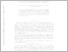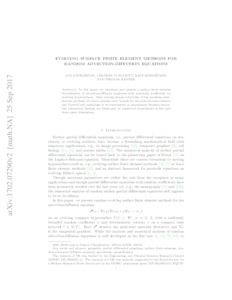Repository: Freie Universität Berlin, Math Department

# Evolving surface finite element methods for random advection-diffusion equations

Djurdjevac, A. and Elliott, C.M. and Kornhuber, R. and Ranner, Th. (2018) Evolving surface finite element methods for random advection-diffusion equations. SIAM/ASA Journal on Uncertainty Quantification, 6 (4). pp. 1656-1684. ISSN 2166-2525Preview

550kB

Official URL: https://doi.org/10.1137/17M1149547

## Abstract

In this paper, we introduce and analyze a surface finite element discretization of advection-diffusion equations with uncertain coefficients on evolving hypersurfaces. After stating the unique solvability of the resulting semidiscrete problem, we prove optimal error bounds for the semidiscrete solution and Monte Carlo sampling of its expectation in appropriate Bochner spaces. Our theoretical findings are illustrated by numerical experiments in two and three space dimensions.

Item Type: Article Mathematical and Computer Sciences > Mathematics > Numerical Analysis Department of Mathematics and Computer Science > Institute of Mathematics 2111 Ekaterina Engel 27 Sep 2017 07:12 12 Dec 2018 08:11

Repository Staff Only: item control page